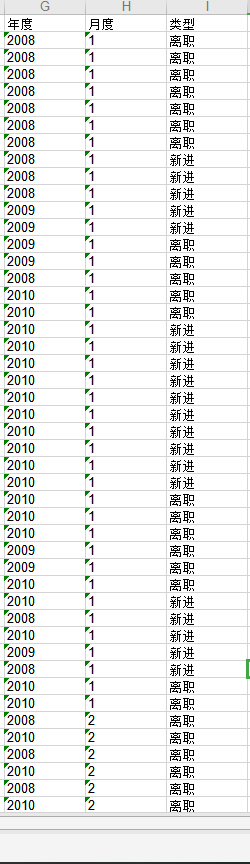2021-01-21 19:06

# SQL查询2009年度，每个月离职的人数，以及环比增长率• 写回答
• 好问题 提建议
• 关注问题
• 收藏
• 邀请回答

#### 1条回答默认 最新

•木子雷 2021-01-21 20:28
已采纳

写好的sql，希望对你有帮助：（sql中使用到的表和字段在下面）

``````-- 查询的2009年最早一个月份的离职数据
select d.month, count(*) as leaveNum, (count(*)-count(*)) as leaveRate  from
(select min(month) as month from t_leave where year = 2009 and type = '离职' )d, t_leave e
where e.year = 2009 and type = '离职' and d.month = e.month GROUP BY d.month

union all

-- 这是2009年除最早月份之外的其他月份的离职数据
select c.amonth , c.aleaveNum, ((c.aleaveNum-c.bleaveNum)/c.bleaveNum) as leaveRate from
(
-- 将本月份的离职数据和上个月份的离职数据合并为一条记录，但是这样就会缺少最早一个月份的离职数据，
-- 但是 union all 上面已经提供了最早月份的离职数据了
select a.month as amonth, b.month as bmonth, a.leaveNum as aleaveNum, b.leaveNum as bleaveNum from
(select month, count(*) as leaveNum  from t_leave where year = 2009 and type = '离职' GROUP BY month)a,
(select month, count(*) as leaveNum  from t_leave where year = 2009 and type = '离职' GROUP BY month)b
where a.month = b.month+1
) c
``````

上面用到的表结构和初始化数据如下：

``````-- 建表语句：
create table T_LEAVE
(
YEAR  VARCHAR2(60),
MONTH VARCHAR2(60),
TYPE  VARCHAR2(60)
)

-- 初始化数据：

INSERT INTO   t_leave  (    year ,  month ,  type ) VALUES ( '2008', '1', '离职');
INSERT INTO   t_leave  (    year ,  month ,  type ) VALUES ( '2009', '1', '在职');
INSERT INTO   t_leave  (    year ,  month ,  type ) VALUES ( '2008', '1', '在职');
INSERT INTO   t_leave  (    year ,  month ,  type ) VALUES ( '2009', '1', '离职');
INSERT INTO   t_leave  (    year ,  month ,  type ) VALUES ( '2009', '2', '离职');
INSERT INTO   t_leave  (    year ,  month ,  type ) VALUES ( '2009', '2', '离职');
INSERT INTO   t_leave  (    year ,  month ,  type ) VALUES ( '2009', '3', '离职');
INSERT INTO   t_leave  (    year ,  month ,  type ) VALUES ( '2009', '1', '离职');
``````
已采纳该答案
评论
解决 无用
打赏 举报